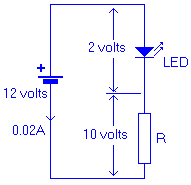## Breaking News

Dropping Resistor Calculator

If you want to try to run something off of a higher recommended voltage their are several ways to do it. The simplest is by a Dropping Resistor. This is useful for simple devices and not more complex electronics which should use the appropriate regulated power adapter to get to the correct voltage. This simple calculator uses the the same basic OHM's law formula as the OHM's Law Calculator but in a more convenient form.

## Real World Example

Lets say you want to hook up a LED (Light Emitting Diode) to 12 Volts. The LED has a specific operating voltage that the manufacturer will tell you and in our case it's 20ma (or 0.02 Amps). The operating voltage is also specified for our particular part as 2.0 Volts. The question becomes what size and value Resistor do I need to safely operate this off of 12 Volts?

What do you know about this problem?

Car Battery Voltage is approx. 12 Volts (nominal, lets stick with this for now)
The LED's Operating Voltage is 2.0 Volts (if you had more then one just add up each voltage, so 3 LED's would use 6 volts)
The LED's Operating Current is 20ma (Milliamps) or 0.02 Amps

We need to find out 2 things, one the Resistance value needed to drop 12 Volts to 2 Volts and the second part of the problem is the wattage rating of the resistor. What is the Wattage Rating? Well when we drop the 12 Volts to 2 Volts we need to get rid of 10 Volts, where does that Voltage go? In the simplest terms HEAT. So we must have a part that can take the heat. Let's Get to it -
Step 1 - Calculate the needed Voltage Drop by subtracting the 2 voltages

Vdrop = 12 Volts - 2 Volts
Vdrop = 10 Volts (this is now the value 'V' in the below Equation)
Step 2 - Calculate the needed Resistance using one of Ohm's Formula

R = V / IWhere :
R = Resistance in Ohms
V = Force in Volts
I = Current in Amps

So the needed Resistance is -
Resistance in Ohms = 10 Volts / 0.02 Amps (Remember this is the same as 20 Milliamps)500 = 10/0.02So what we need is a 500 Ohm Resistor, that was pretty easy. But Resistors come in different sizes based on their Wattage rating. So now lets figure that out.Step 3 - Calculate Resistor Wattage from the Current and Resistance using another of Ohm's Formula
P = I2
Where :P = Power in Watts
I = Current in Amps
R = Resistance in Ohms

So the needed Wattage is -

Power Watts = 0.02 Amps x 0.02 Amps * 500 Ohms
0.2 = 0.02 x 0.02 x 500

The formula tells us that we are using 0.2 Watts of power and the resistor should be larger then that to survive the heat. Typical sizes for small resistors are 1/8 Watt, 1/4 Watt, 1/2 Watt, 1 Watt, and so on. So in our case a 1/4 Watt (0.25) might be on the small size, but a 1/2 (0.5) Watt resistor should be fine. Using a larger Wattage resistor is almost always OK.